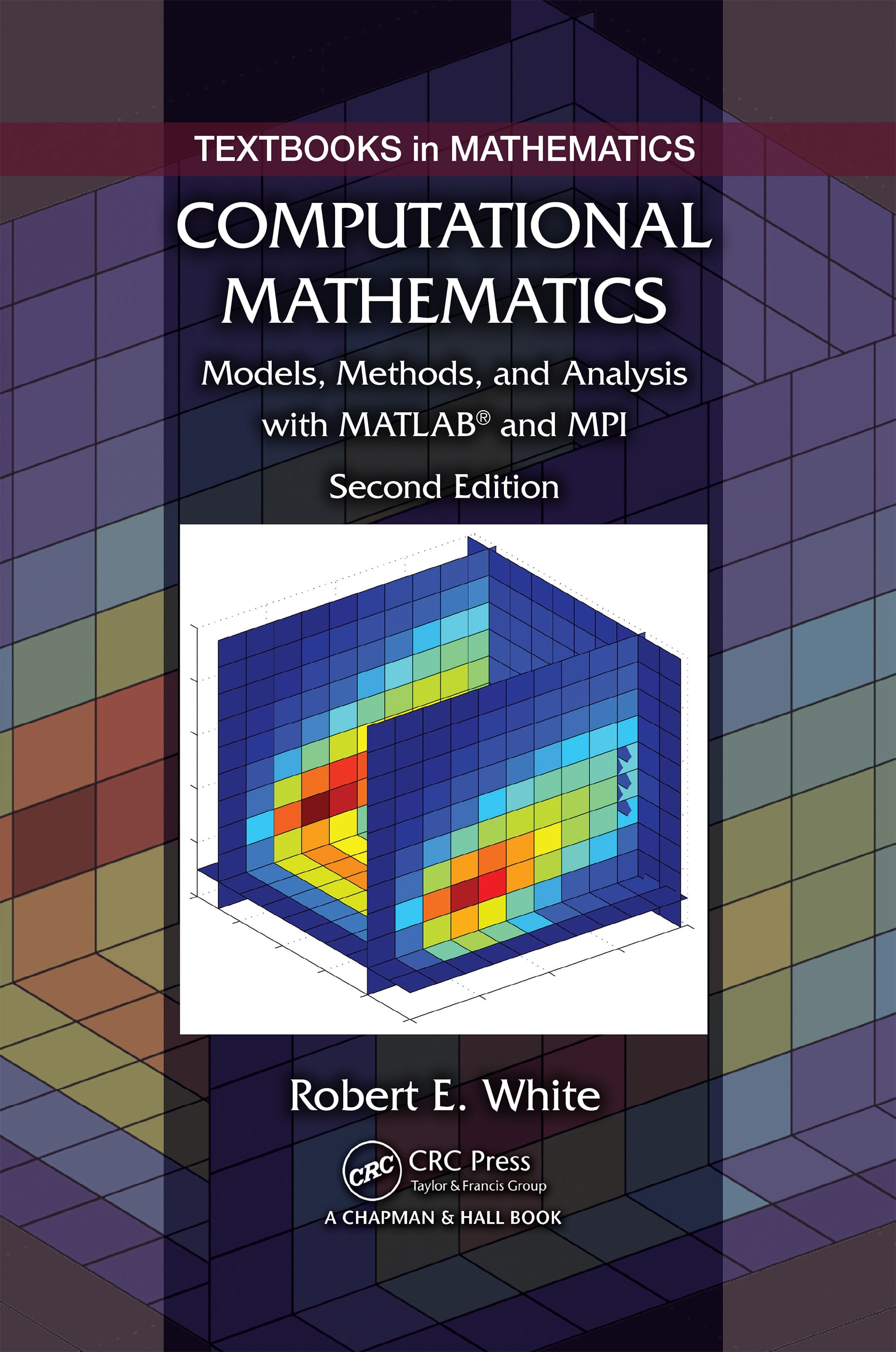# Computational Mathematics

## Models, Methods, and Analysis with MATLAB® and MPI, Second Edition, 2nd Edition

Chapman and Hall/CRC

478 pages | 97 B/W Illus.

##### Purchasing Options:\$ = USD
Hardback: 9781482235159
pub: 2015-11-17
SAVE ~\$24.00
\$120.00
\$96.00
x
eBook (VitalSource) : 9780429160301
pub: 2015-11-11
from \$60.00

FREE Standard Shipping!

### Description

Computational Mathematics: Models, Methods, and Analysis with MATLAB® and MPI is a unique book covering the concepts and techniques at the core of computational science. The author delivers a hands-on introduction to nonlinear, 2D, and 3D models; nonrectangular domains; systems of partial differential equations; and large algebraic problems requiring high-performance computing. The book shows how to apply a model, select a numerical method, implement computer simulations, and assess the ensuing results.

Providing a wealth of MATLAB, Fortran, and C++ code online for download, the Second Edition of this very popular text:

• Includes a new chapter with two sections on the finite element method, two sections on shallow water waves, and two sections on the driven cavity problem
• Introduces multiprocessor/multicore computers, parallel MATLAB, and message passing interface (MPI) in the chapter on high-performance computing
• Updates and adds code and documentation

Computational Mathematics: Models, Methods, and Analysis with MATLAB® and MPI, Second Edition is an ideal textbook for an undergraduate course taught to mathematics, computer science, and engineering students. By using code in practical ways, students take their first steps toward more sophisticated numerical modeling.

Discrete Time–Space Models

Newton Cooling Models

Heat Diffusion in a Wire

Diffusion in a Wire with Little Insulation

Flow and Decay of a Pollutant in a Stream

Heat and Mass Transfer in Two Directions

Convergence Analysis

Steady-State and Triangular Solves

Heat Diffusion and Gauss Elimination

Cooling Fin and Tridiagonal Matrices

Schur Complement

Convergence to Continuous Model

Poisson Equation Models

Steady-State and Iterative Methods

Heat Transfer in 2D Fin and SOR

Fluid Flow in a 2D Porous Medium

Ideal Fluid Flow

Deformed Membrane and Steepest Descent

Nonlinear and 3D Models

Nonlinear Problems in One Variable

Nonlinear Heat Transfer in a Wire

Nonlinear Heat Transfer in 2D

Steady-State 3D Heat Diffusion

Time-Dependent 3D Diffusion

High-Performance Computations in 3D

FEM and Fluid Flows

FEM and Linear Shape Functions

FEM with Heat and Fluid Flow

Lax–Wendroff Methods

Shallow Water Wave Model

Navier–Stokes Equations

Discrete Navier–Stokes Equations

Epidemics, Images, and Money

Epidemics and Dispersion

Epidemic Dispersion in 2D

Image Restoration

Restoration in 2D

Option Contract Models

Black—Scholes Model for Two Assets

High-Performance Computing

CPUs and Vector Pipes

Multiprocessor and Multicore Computers

Parallel MATLAB

Parallel MATLAB and SPMD

Introduction to MPI

MPI and Matrix Products

Message Passing Interface

Collective Subroutines

Grouped Datatypes

Communicators

Fox Algorithm for AB

Shared Memory and OpenMP

Hybrid MPI/OpenMP

Classical Methods for Ax = d

Gauss Elimination

Symmetric Positive Definite Matrices

Domain Decomposition and MPI

SOR and P-Regular Splittings

SOR and MPI

Krylov Methods for Ax = d

Preconditioners

PCG and MPI

Least Squares

GMRES

GMRES(m) and MPI

### Subject Categories

##### BISAC Subject Codes/Headings:
MAT000000
MATHEMATICS / General
MAT021000
MATHEMATICS / Number Systems